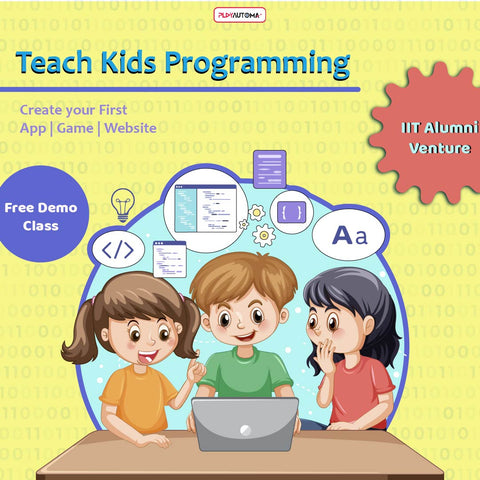Free Shipping PAN India | Get 15% off with code PLAYAUTOMA15 on orders above ₹999

# Java CurriculumModule 1 covers basic concepts of Java i.e. Variables, Data Types, Input/Output, Conditional statements, Loop Statements etc.

Module 2 includes Advanced Java Programming like Arrays, String Handling, Inheritance, Encapsulation.

Detailed structure is as follows:

Module 1: Java Basic Concepts (24 hours)

1. Overview Of Java Platform

• Setting JDK

• An Introduction - java, javac, bytecode, JDK, JVM

• Explanation of Main Method

• Compilation of Java Program

• Different Type of Errors : Logical, Syntax and Run-Time

• Print the Output - System.out.println() , System.out.print()

• Write first Java Program.

• Introduction to Packages and Import Statement

2. Variable, Data Type and Operators

• Variables and their Data Types - primitive types and non-primitive types, primitive types with their size, Unicode and ASCII, escape sequences, local and global variables.

• Literals

• Program to Swap the values.

• Input in Java - Using methods of Scanner class [nextShort( ), nextInt( ), nextLong( ), nextFloat ( ), nextDouble( ), next( ), nextLine( ), next ( ) .charAt(0) ]

• Type Conversion - Implicit and Explicit

• Program to Compute Quotient and Remainder

• Operators - Type of Operators (Arithmetic, Relational, Logical, Assignment), precedence and associativity of operators, prefix and postfix.

• Expressions

• Program to calculate Simple Interest .

3. Control Statement

• IF Statement

• Program to check if the number is two digit number .

• IF ELSE Statement

• Program to check if the no. is odd or even.

• IF ELSE IF Ladder Statement

• Program to check divisibility of a number.

• Nested IF Statement

• Program to find eligibility to vote in election.

• Ternary Operator

• Program to check if the number is positive or negative.

4. Working with different Loops

• For Loops

• Program to find the sum of all digits of a 5-digit number.

• Nested For loop

• Program to Print a Pattern.

• While Loops

• Program to Find Reverse of a Number.

• Do While Loop

• Program to display all the prime numbers between 1-100.

• Infinite WHILE Loop

• Delay Loop

• Jump Statements - Break and Continue

• Program to check if the given year is a Leap Year or not.

• Switch Statement –

• Program to check Vowel or Consonant.

Module 2: Concepts of Object Oriented Programming (24 hours)

1. Introduction to Object Oriented Programming

2. Access Specifiers - Visibility rules for private, protected and public access specifiers. Scope of variables, class variables, instance variables, argument variables .

3. Java Class and object

• Class as an object factory

• Pre-defined classes

• User defined classes

• Objects as instances of a class

• Temporary instances

• Program to create a class and objects .

3. User Defined Methods

• Without Parameters

• With Parameters

• Return Types

• Call by value

• Call by Reference

• Program to display the HCF and LCM of two numbers.

• Static and Non-Static Methods

4. Polymorphism

• Program to calculate the area and perimeter of different figures.

5. Encapsulation

6. Inheritance

• Program to create the subclass of a class using inheritance .

7. Constructor

• Default constructor

• Parameterized Constructor

• Program to print the record of a student

• Difference between constructor and method

• Program to calculate volume of 3-D figures .

8. Library Classes

• Autoboxing and Unboxing

• Wrapper classes - int parseInt(String s), long parseLong(String s), float parseFloat(String s), double parseDouble(String s), boolean isDigit(char ch), boolean isLetter(char ch), boolean isLetterOrDigit(char ch), boolean isLowerCase(char ch), boolean isUpperCase(char ch), boolean isWhitespace(char ch), char toLowerCase (char ch) , char toUpperCase(char ch)

• Program to check whether a given character is an uppercase/ lowercase / digit.

9. Arrays

• Single and Double Dimensional Arrays

• Program to access the elements of an array.

• Sorting techniques - selection sort and bubble sort

• Program to sort an array in increasing order using selection sort.

• Program to sort an array of strings using bubble sort

• Search techniques - linear search and binary search

• Program to search a particular number in an array using binary search.

• Program to search a customer’s name in a hotel’s record using linear search

10. String Handling

• Methods of String Class

• String Array

• String as Array of Characters

• Program to count the total number of words in a sentence .

• Concatenation

• Program to remove all the vowels of a sentence.

11. Mathematical Library Functions - pow(x,y), sqrt(x), cbrt(x), ceil(x), floor(x), round (x), abs(a), max(a, b), min(a,b), random( ) .

Program to find the roots of a quadratic equation.

Note:

• Courses can be taken module-wise

• Class Test and assignments will be held after each module

• Age : 12+